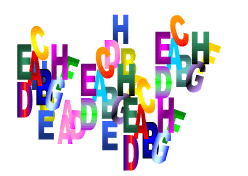#### You may also like### Not a Polite Question

When asked how old she was, the teacher replied: My age in years is not prime but odd and when reversed and added to my age you have a perfect square...### Whole Numbers Only

Can you work out how many of each kind of pencil this student bought?### Symmetricality

Add up all 5 equations given below. What do you notice? Solve the system and find the values of a, b, c , d and e. b + c + d + e = 4 a + c + d + e = 5 a + b + d + e = 1 a + b + c + e = 2 a + b + c + d = 0

# Letter Land

##### Age 11 to 14Challenge Level

If$A + C = A$

$F \times D = F$

$B - G = G$

$A + H = E$

$B / H = G$

$E - G = F$

and $A$-$H$ represent the numbers from $0$ to $7$

Find the values of $A, B, C, D, E, F, G$ and $H$.